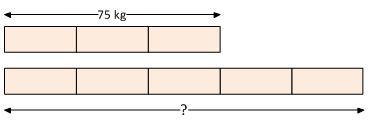### Sample Problem

The total weight of 3 identical bags of rice is 75 kg. What is the total weight of 5 such bags of rice?

kg

#### SolutionThe weight of each bag of rice: 75 kg ÷ 3 = 25 kg

Thus, the total weight of 5 bags of rice: 25 kg × 5 = 125 kg